# Activity in WPF Diagram (SfDiagram)

5 May 202117 minutes to read

The `Activity` is a task that is performed in a business process. It is represented by a rounded rectangle.

There are two types of activities. They are listed as follows:

• Task: Occurs within a process and it is not broken down to a finer level of detail.
• CollapsedSubProcess: Occurs within a process and it is broken down to a finer level of detail.

You can specify the any one of the above activity type using the ActivityType property of `BpmnNodeViewModel.`

``````<!--Initialize the SfDiagram-->
<syncfusion:SfDiagram x:Name="diagram">
<!--Initialize the Node-->
<syncfusion:SfDiagram.Nodes>
<!--Initialize the Node Collection-->
<syncfusion:NodeCollection>
<!--Initialize the BpmnNodeViewModel-->
<syncfusion:BpmnNodeViewModel UnitHeight="70" UnitWidth="100" OffsetX="100" OffsetY="100" Type="Activity" ActivityType="Task">
</syncfusion:BpmnNodeViewModel>
</syncfusion:NodeCollection>
</syncfusion:SfDiagram.Nodes>
</syncfusion:SfDiagram>``````
``````//Initialize the diagram.
SfDiagram diagram = new SfDiagram();

//Initialize the BpmnNodeViewModel.
BpmnNodeViewModel node = new BpmnNodeViewModel()
{
OffsetX = 100,
OffsetY = 100,
UnitHeight = 70,
UnitWidth = 100,
Type = BpmnShapeType.Activity,
};

// Add the node into the Node's collection.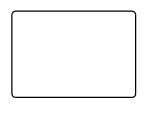The `TaskType` property of the `BpmnNodeViewModel` allows you to define the type of task such as sending, receiving, user-based task, and more. By default, the TaskType property of the task is set to None. This is shown by a small event symbol in the top of the corner. The following code explains how to create different types of BPMN tasks.

``````<!--Initialize the SfDiagram-->
<syncfusion:SfDiagram x:Name="diagram">
<!--Initialize the Node-->
<syncfusion:SfDiagram.Nodes>
<!--Initialize the Node Collection-->
<syncfusion:NodeCollection>
<!--Initialize the BpmnNodeViewModel-->
</syncfusion:BpmnNodeViewModel>
</syncfusion:NodeCollection>
</syncfusion:SfDiagram.Nodes>
</syncfusion:SfDiagram>``````
``````//Initialize the diagram.
SfDiagram diagram = new SfDiagram();

//Initialize the BpmnNodeViewModel.
BpmnNodeViewModel node = new BpmnNodeViewModel()
{
OffsetX = 100,
OffsetY = 100,
UnitHeight = 70,
UnitWidth = 100,
Type = BpmnShapeType.Activity,
};

// Add the node into the Node's collection.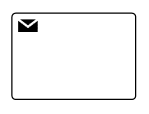The various types of BPMN tasks are tabulated as follows.

Service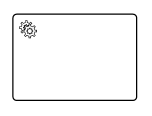A Service task is a task that uses a web service, an automated application, or other kinds of service in completing the task
SendA Send task is represents a task that sends a message from one to another. The task is completed once the message has been sent
Receive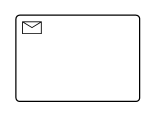A Receive task indicates the wait for the arrival of a certain message. The task is completed once the message has been received
Instantiating Receive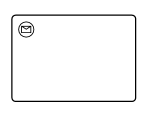A receive task is used to instantiate a process that is the receive task replace the message start event
Manual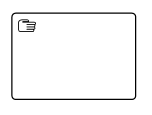A Manual task is a task that is performed without the aid of any business process execution engine or any application
Business Rule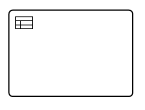A Business Rule task is used to synchronously execute one or more rules
User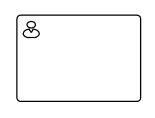A User task represents that a human performer performs the task with the use of a software application
Script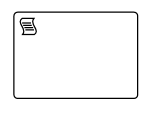A Script task is an automated activity when a process execution arrives at the Script task, the corresponding script is executed

## BPMN activity sub process

A `CollapsedSubProcess` is a group of tasks that is used to hide or reveal details of additional levels. The following code explains how to create CollapsedSubProcess.

``````<!--Initialize the SfDiagram-->
<syncfusion:SfDiagram x:Name="diagram">
<!--Initialize the Node-->
<syncfusion:SfDiagram.Nodes>
<!--Initialize the Node Collection-->
<syncfusion:NodeCollection>
<!--Initialize the BpmnNodeViewModel-->
<syncfusion:BpmnNodeViewModel UnitHeight="70" UnitWidth="100" OffsetX="100" OffsetY="100" Type="Activity" ActivityType="CollapsedSubProcess" >
</syncfusion:BpmnNodeViewModel>
</syncfusion:NodeCollection>
</syncfusion:SfDiagram.Nodes>
</syncfusion:SfDiagram>``````
``````//Initialize the diagram.
SfDiagram diagram = new SfDiagram();

//Initialize the BpmnNodeViewModel.
BpmnNodeViewModel node = new BpmnNodeViewModel()
{
OffsetX = 100,
OffsetY = 100,
UnitHeight = 70,
UnitWidth = 100,
Type = BpmnShapeType.Activity,
ActivityType=ActivityType.CollapsedSubProcess,
};

// Add the node into the Node's collection.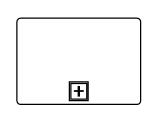### Loop

`LoopActivity` is a task that is internally being looped. The LoopActivity property of BpmnNodeViewModel allows you to define the type of loop. The default value for the `LoopActivity` is None.

You can define the LoopActivity in both tasks and collapsed subprocess of the Activity.

``````<!--Initialize the SfDiagram-->
<syncfusion:SfDiagram x:Name="diagram">
<!--Initialize the Node-->
<syncfusion:SfDiagram.Nodes>
<!--Initialize the Node Collection-->
<syncfusion:NodeCollection>
<!--Initialize the BpmnNodeViewModel-->
<syncfusion:BpmnNodeViewModel UnitHeight="70" UnitWidth="100" OffsetX="100" OffsetY="100" Type="Activity" ActivityType="Task" LoopActivity="Standard" >
</syncfusion:BpmnNodeViewModel>
</syncfusion:NodeCollection>
</syncfusion:SfDiagram.Nodes>
</syncfusion:SfDiagram>``````
``````//Initialize the diagram.
SfDiagram diagram = new SfDiagram();

//Initialize the BpmnNodeViewModel.
BpmnNodeViewModel node = new BpmnNodeViewModel()
{
OffsetX = 100,
OffsetY = 100,
UnitHeight = 70,
UnitWidth = 100,
Type = BpmnShapeType.Activity,
LoopActivity = LoopCharacteristic.Standard
};

// Add the node into the Node's collection.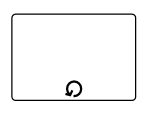The following table contains various types of BPMN loops.

None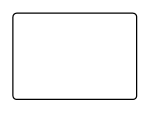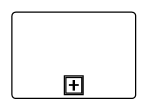None of the shape shows in the sub-process
Standard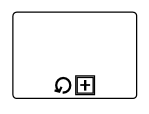Loop marker indicates that the sub-process repeats itself in the sequence
SequenceMultiInstance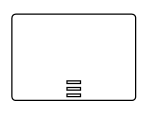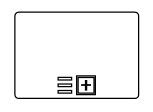Multi-Instance marker indicates that the sub-process can run with other identical sub-processes simultaneously. The three horizontal lines indicate the sequential execution
ParallelMultiInstance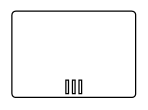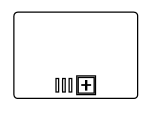Multi-Instance marker indicates that the sub-process can run with other identical sub-processes simultaneously. The three vertical lines indicate that the instances will be executed in parallel

### Compensation

Compensation is triggered when the operation is partially failed and enabled it with the compensation property in both task and the collapsed subprocess. To create a compensation, you have to enable the IsCompensationActivity property of the BPMNNodeViewModel.

Note: By default, the IsCompensationActivity property is false.

``````<!--Initialize the SfDiagram-->
<syncfusion:SfDiagram x:Name="diagram">
<!--Initialize the Node-->
<syncfusion:SfDiagram.Nodes>
<!--Initialize the Node Collection-->
<syncfusion:NodeCollection>
<!--Initialize the BpmnNodeViewModel-->
<syncfusion:BpmnNodeViewModel UnitHeight="70" UnitWidth="100" OffsetX="100" OffsetY="100" Type="Activity" ActivityType="Task" IsCompensationActivity="True" >
</syncfusion:BpmnNodeViewModel>
</syncfusion:NodeCollection>
</syncfusion:SfDiagram.Nodes>
</syncfusion:SfDiagram>``````
``````//Initialize the diagram.
SfDiagram diagram = new SfDiagram();

//Initialize the BpmnNodeViewModel.
BpmnNodeViewModel node = new BpmnNodeViewModel()
{
OffsetX = 100,
OffsetY = 100,
UnitHeight = 70,
UnitWidth = 100,
Type = BpmnShapeType.Activity,
IsCompensationActivity = true
};

// Add the node into the Node's collection.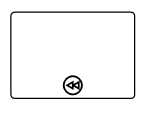### Call

A Call activity is a global sub-process that is reused at various points of the business flow. To create a Call activity, you have to enable the IsCallActivity property of the BPMNNodeViewModel.

Note: By default, the IsCallActivity property is false.

``````<!--Initialize the SfDiagram-->
<syncfusion:SfDiagram x:Name="diagram">
<!--Initialize the Node-->
<syncfusion:SfDiagram.Nodes>
<!--Initialize the Node Collection-->
<syncfusion:NodeCollection>
<!--Initialize the BpmnNodeViewModel-->
<syncfusion:BpmnNodeViewModel UnitHeight="70" UnitWidth="100" OffsetX="100" OffsetY="100" Type="Activity" ActivityType="Task" IsCallActivity="True" >
</syncfusion:BpmnNodeViewModel>
</syncfusion:NodeCollection>
</syncfusion:SfDiagram.Nodes>
</syncfusion:SfDiagram>``````
``````//Initialize the diagram.
SfDiagram diagram = new SfDiagram();

//Initialize the BpmnNodeViewModel.
BpmnNodeViewModel node = new BpmnNodeViewModel()
{
OffsetX = 100,
OffsetY = 100,
UnitHeight = 70,
UnitWidth = 100,
Type = BpmnShapeType.Activity,
IsCallActivity = true
};

// Add the node into the Node's collection.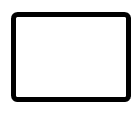An ad-hoc subprocess is a group of tasks that are executed in any order or skipped in order to fulfill the end condition. To create a ad-hoc activity, you have to enable the `IsAdhocActivity` property of the BPMNNodeViewModel.

Note: By default, the IsAdhocActivity property is false.

``````<!--Initialize the SfDiagram-->
<syncfusion:SfDiagram x:Name="diagram">
<!--Initialize the Node-->
<syncfusion:SfDiagram.Nodes>
<!--Initialize the Node Collection-->
<syncfusion:NodeCollection>
<!--Initialize the BpmnNodeViewModel-->
<syncfusion:BpmnNodeViewModel UnitHeight="100" UnitWidth="100" OffsetX="100" OffsetY="100" Type="Activity" ActivityType="CollapsedSubProcess" IsAdhocActivity="True" >
</syncfusion:BpmnNodeViewModel>
</syncfusion:NodeCollection>
</syncfusion:SfDiagram.Nodes>
</syncfusion:SfDiagram>``````
``````//Initialize the diagram.
SfDiagram diagram = new SfDiagram();

//Initialize the BpmnNodeViewModel.
BpmnNodeViewModel node = new BpmnNodeViewModel()
{
OffsetX = 100,
OffsetY = 100,
UnitHeight = 100,
UnitWidth = 100,
Type = BpmnShapeType.Activity,
ActivityType = ActivityType.CollapsedSubProcess,
};

// Add the node into the Node's collection.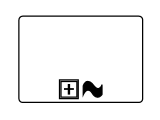### SubProcessType

SubProcessType represents the type of task that is being processed. The `SubProcessType` property of BpmnNodeViewModel allows you to define the type of SubProcess. By default, it is set to Default.

``````<!--Initialize the SfDiagram-->
<syncfusion:SfDiagram x:Name="diagram">
<!--Initialize the Node-->
<syncfusion:SfDiagram.Nodes>
<!--Initialize the Node Collection-->
<syncfusion:NodeCollection>
<!--Initialize the BpmnNodeViewModel-->
<syncfusion:BpmnNodeViewModel UnitHeight="100" UnitWidth="100" OffsetX="100" OffsetY="100" Type="Activity" ActivityType="CollapsedSubProcess" SubProcessType="Event" >
</syncfusion:BpmnNodeViewModel>
</syncfusion:NodeCollection>
</syncfusion:SfDiagram.Nodes>
</syncfusion:SfDiagram>``````
``````//Initialize the diagram.
SfDiagram diagram = new SfDiagram();

//Initialize the BpmnNodeViewModel.
BpmnNodeViewModel node = new BpmnNodeViewModel()
{
OffsetX = 100,
OffsetY = 100,
UnitHeight = 100,
UnitWidth = 100,
Type = BpmnShapeType.Activity,
ActivityType = ActivityType.CollapsedSubProcess,
SubProcessType = SubProcessType.Event
};

// Add the node into the Node's collection.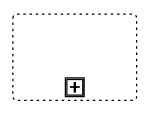Call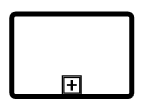It is a global sub-process that is reused at various points in the business flow
EventThe event sub-process is a sub-process that is triggered by an event. An event sub-process can be added at the process level or at any sub-process level
DefaultThe task that is performed in a business process. It is represented by a rounded rectangle
Transaction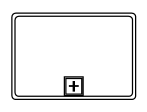It is a specialized sub-process that involves payment